# Average Values

 Quiz Answers: (1) 29, (2)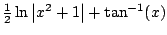Exam 1: Wednesday, Feb 1, 7:00pm-7:50pm, here. Today: §6.5 - Average Values Today: §10.3 - Polar coords NEXT: §10.4 -Areas in Polar coords Why did we skip from §6.5 to §10.3? Later we'll go back and look at trig functions and complex exponentials; these ideas will fit together more than you might expect. We'll go back to §7.1 on Feb 3.

In this section we use Riemann sums to extend the familiar notion of an average, which provides yet another physical interpretation of integration.

Recall: Suppose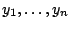are the amount of rain each day in La Jolla, since you moved here. The average rainful per day is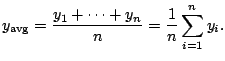Definition 3.3.1 (Average Value of Function)   Suppose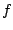is a continuous function on an interval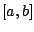. The average value ofonis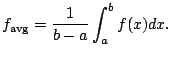Motivation: If we sampleat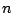points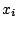, then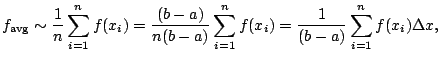since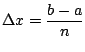. This is a Riemann sum!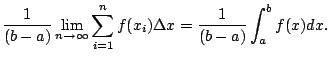This explains why we defined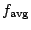as above.

Example 3.3.2   What is the average value of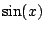on the interval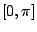?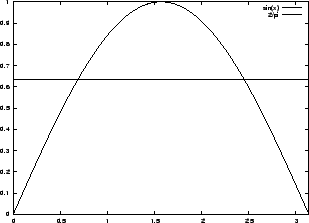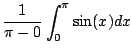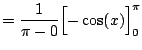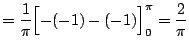Observation: If you multiply both sides by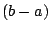in Definition 3.3.1, you see that the average value times the length of the interval is the area, i.e., the average value gives you a rectangle with the same area as the area under your function. In particular, in Figure 3.3.1 the area between the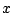-axis andis exactly the same as the area between the horizontal line of height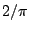and the-axis.

Example 3.3.3   What is the average value ofon the interval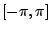?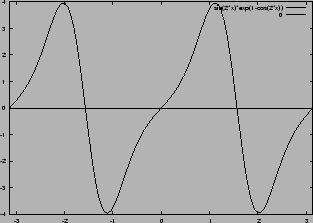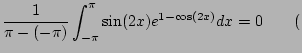since the function is odd!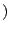Theorem 3.3.4 (Mean Value Theorem)   Supposeis a continuous function on. Then there is a number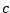insuch that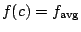.

This says thatassumes its average value. It is a used very often in understanding why certain statements are true. Notice that in Examples 3.3.2 and 3.3.3 it is just the assertion that the graphs of the function and the horizontal line interesect.

Proof. Let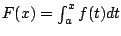. Then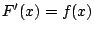. By the mean value theorem for derivatives, there is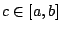such that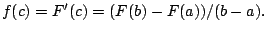But by the fundamental theorem of calculus,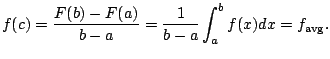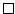William Stein 2006-03-15